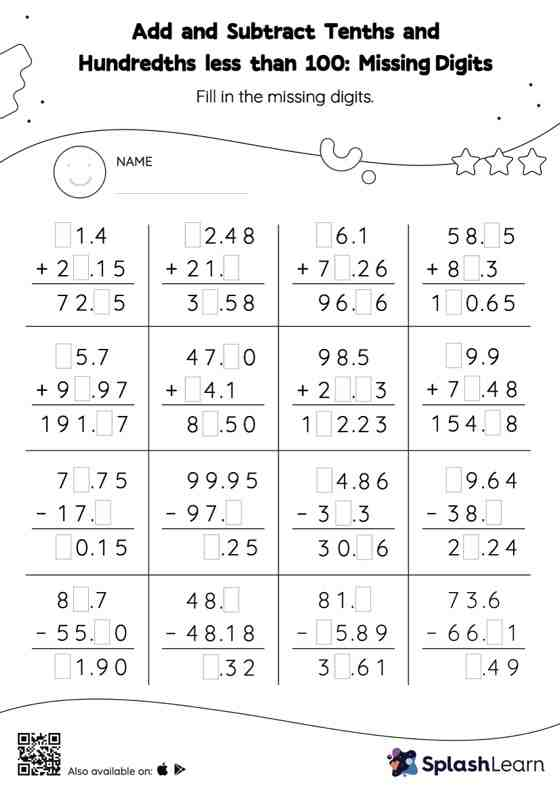# Add and Subtract Tenths and Hundredths less than 100: Missing Digits Worksheet

Home > Add and Subtract Tenths and Hundredths less than 100: Missing DigitsTask your little mathematicians to crack the code of adding and subtracting tenths and hundredths less than 100 with this fun worksheet. Students align the decimal points and use zero as space holders to add and subtract decimals. Then they use the relationship between addition and subtraction to find the missing number in add and subtract tenths and hundredths less than 100 worksheet.